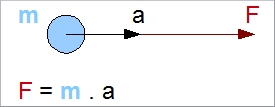# Newton's Laws (1st, 2nd and 3rd)

## Newton's First Law

The First Law is formulated as follows:

A particle will stay at rest or continue at a constant velocity unless acted upon by an external unbalanced force.

### Explanation

Every object that has no external force working on it will not change its state. If it was at rest it will stay at rest; if it was travelling, then it will continue to travel at the same velocity and in the same direction. Nothing changes in this respect when there is no force acting upon it. Note the use of the word unbalanced in the law; balanced forces cancel each other out, with the net result being no force.

### Examples

A helicopter in a hover (a few feet above the ground) is an example of an object that is at rest. In the horizontal plane, there are no forces acting upon it. In the vertical plane, the forces are balanced: weight versus vertical thrust. The helicopter stays at rest.

Another, more theoretical example: assume that there is no friction/drag involved; you have the same hovering helicopter, but it is now being given a push by someone (don't try this at home!) The helicopter will begin to move in the direction it is being pushed, and will continue to do so. It will not stop. In reality, however, the helicopter will stop moving because another force is working on it: friction; in this case, better known as drag.

## Newton's Second Law

F = m . a

where:
F = Force (N)
m = Mass (kg)
a = Acceleration (m/s2)

The net force on an object is equal to the mass of the object multiplied by its acceleration.### Explanation

This law states that the force needed to accelerate an object is proportional to its mass. The greater the object's mass, the greater the force required (for the same amount of acceleration). Note that this law is sometimes referred to as the law of Inertia. The mass of an object is a measure of its inertia (inertia is the property of an object to resist a change in movement).

### Examples

Assuming that the weight and vertical thrust remain the same, the helicopter from the previous example now wants to move forward. In order to do so, it must accelerate by applying a forward directed force. It will then start to move. Put more precisely, it will accelerate by the amount of F/m. The smaller the mass of the helicopter, the greater the acceleration (with the same amount of force applied). Acceleration will occur as long as the force is applied (assuming that there is no friction and drag). When the forward force is removed, the helicopter will travel forwards at the speed achieved and will continue its journey at that velocity.

We will now consider the helicopter which is still moving at a constant speed (as there is no forward force being applied to it), and take into account the effect of drag (friction caused by moving through the air). The helicopter will decelerate as a result of the drag, which is acting in the opposite direction. This deceleration will continue until the helicopter comes to a rest in the air; the forces of drag will act for as long as there is drag involved (drag exists as long as there is air movement).

Note that the time it takes to achieve zero speed depends on the initial speed, the mass of the helicopter and the amount of drag. A helicopter with more mass will take longer to arrive at zero speed (assuming the same amount of drag).

## Newton's Third Law

This Law is stated as follows:

Every action has an equal and opposite reaction.

### Explanation

This Law states that when there is a force working on an object, there is always an equal force in the opposite direction. This force is not, however, working on the same object (so the two forces can never cancel each other out).

### Examples

When a car stops, there are forces in play between the wheels and the surface of the road. There is a force working on each wheel, and there are opposite forces of the same magnitude working on the road’s surface.
When an airfoil is moving through the air, there are friction forces (drag) working on it. Forces of the same magnitude, and in the opposite direction, are working on the air.
When a helicopter lands on a helipad, its weight is borne by the skids. There is a force working on the skids to hold the weight of the helicopter. An equal and opposite force is working on the helipad’s surface.

A classic example in the helicopter domain is the torque applied by the engine to rotate the main shaft (with the rotors attached). The third law states that there must be an equal torque in the opposite direction. The first force (torque) is acting on the shaft, and the second (reaction) is working on the engine. This means that when the engine is running, the main shaft will turn in one direction and the engine in the opposite one. Because the engine is connected to the fuselage, the fuselage will start rotating because it is not fixed but 'hanging' in free air. The tail boom rotor (anti torque rotor) exists to prevent rotation of the fuselage, and provides a force with which to stop this from happening.

# Do you want to comment this topic?

1: (Book) Cyclic and Collective2: (Book) Principles of Helicopter Flight3: Microsoft FSX Steam Edition4: Logitech Extreme 3D Pro Joystick5: Saitek Pro Flight Rudder Pedals